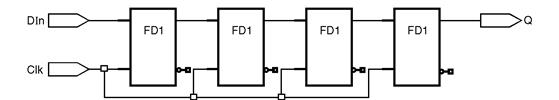Home > VHDL > Shift Registers > 4 bit shift register

Shift-Register

The main usage for a shift register is for converting from a serial data input stream to a parallel data output or vice versa. For a serial to parallel data conversion, the bits are shifted into the register at each clock cycle, and when all the bits (usually eight bits) are shifted in, the 8-bit register can be read to produce the eight bit parallel output. For a parallel to serial conversion, the 8-bit register is first loaded with the input data. The bits are then individually shifted out, one bit per clock cycle, on the serial output line. In general a shift register is characterized by the following control and data signals:

• Clock
• Serial input
• Asynchronous set/reset
• Synchronous set/reset
• Clock enable

* Serial or parallel output. The shift register output mode may be:

1. Serial : only the contents of the last flip-flop is accessed by the rest of the
circuit.

2. Parallel : the contents of one or several of flip-flops other than the last one, is
accessed

• Shift modes: left, right, etc.4 bit shift register :

Fig. 6.12.1 : 4 bit Shift register

library IEEE;

use IEEE.std_logic_1164.all;

entity ShiftRegister is

port ( DIn, Clk : in std_logic;

Q : out std_logic);

end ShiftRegister;

architecture Example of ShiftRegister is

begin

process (Clk, DIn)

variable DTmp : std_logic_vector(3 downto 0);

begin

if Clk'event and Clk = '1' then

DTmp := DTmp(2 downto 0) & DIn;

Q <= DTmp(3);

end if;

end process;

end Example;

1) 4-bit shift-left/shift-right register with positive-edge clock, serial in, and
parallel out :

In this model one set the direction of shift when mode selector left_right signal is '0' then input signal shifted to left when it is '1' its direction is right.

library ieee;

use ieee.std_logic_1164.all;

entity shift_lr is

port (Clock, Sin, left_right : in std_logic;

Pout : out std_logic_vector(3 downto 0));

end shift_lr;

architecture exam of shift_lr is

signal temp: std_logic_vector(3 downto 0);

begin

process (Clock)

begin

if (Clock'event and Clock='1') then

if (left_right='0') then

temp <= temp(2 downto 0) & Sin;

else

temp <= Sin & temp(3 downto 1);

end if ;

end if ;

end process;

Pout <= temp;

end exam;

2) 4 bit, positive clock, synchronous set & clear, loadable parallel in â€“ parallel
out (PIPO) shift register :

library IEEE;

use IEEE.std_logic_1164.all;

entity shft_reg is

port ( CLK : in std_logic; -- Clock input CLK active high

CE : in std_logic; -- Clock enable input CE active high

CLR : in std_logic; -- Synchronous Clear input CLR active high

SET : in std_logic; -- Synchronous Set input SET active high

DATA : in std_logic_vector(3 downto 0);

O : out std_logic_vector(3 downto 0));

end shft_reg;

architecture shft_reg_arch of shft_reg is

signal TEMP_O : std_logic_vector(3 downto 0);

begin

process(CLK)

begin

if rising_edge(CLK) then

if CE = '1' then

if CLR = '1' then

TEMP_O <= (others => '0');

elsif SET = '1' then

TEMP_O <= (others => '1');

TEMP_O <= DATA;

else

TEMP_O <= '0' & TEMP_O(3 downto 1);

end if ;

end if ;

end if;

end process;

O <= TEMP_O;

end architecture;